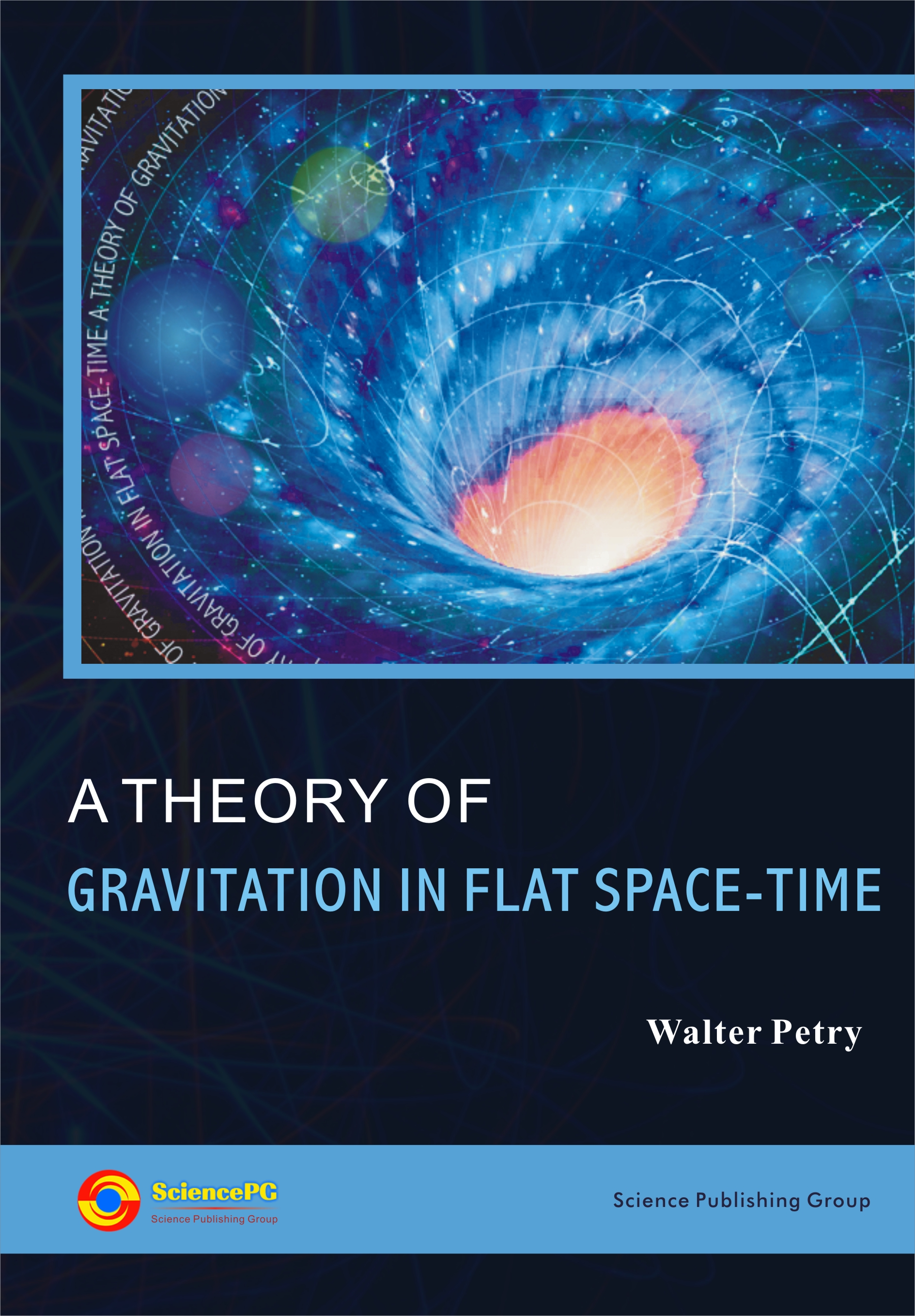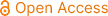A Theory of Gravitation in Flat Space-Time

ISBN: 978-1-940366-11-1
Published Date: April, 2014
Pages: 182
Paperback: \$89
Publisher: Science Publishing Group
 To purchase hard copies of this book, please email: book@sciencepublishinggroup.com
Book Description

The theory of gravitation uses a flat space-time metric, e.g., the pseudo-Euclidean geometry. The gravitational field is described by a tensor of order 2 (potentials) which satisfies covariant (relative to the metric) differential equations of order 2. The left hand side of the differential equations is the total energy-momentum tensor of matter and the gravitational field. The theory is a field theory in analogy to the equations of Maxwell for Electrodynamics and not a geometrical description of gravitation. Applications: The results of the theory agree for weak gravitational field with those of general relativity to the measurable order but for strong gravitational fields are differences, e.g. in the beginning of the universe. The space is flat by the theory. There are non-singular cosmological models, i.e., there is no big bang but the universe may be very hot in the beginning. The universe contracts to a small positive value and then it expands for all times. A new interpretation of a non-expanding universe can also be given. A modified Hubble law is also received in agreement with the results of Arp who states that there are in the universe nearby quasars with quite different redshifts. In addition, post- Newtonian approximations in the matter dominated universe can give large density contrasts. This is important to explain the large scale-structures in the universe, e.g. galaxies, etc. We need no inflation because the space is flat and not curved.

Author Introduction

Walter Petry, Mathematisches Institut der Universitaet Duesseldorf, Duesseldorf, Germany

• The Whole Book

• Front Matter

• Chapter 1 Theory of Gravitation

1. 1.1 Gravitational Potentials
2. 1.2 Lagrangian
3. 1.3 Field Equations
4. 1.4 Equations of Motion and the Energy-Momentum
5. 1.5 Field Equations Rewritten
6. 1.6 Field Strength and Field Equations
7. 1.7 Angular-Momentum
8. 1.8 Equations of the Spin Angular Momentum
9. 1.9 Transformation to Co-Moving Frame
10. 1.10 Approximate Solution in Empty Space
• Chapter 2 Static Spherical Symmetry

1. 2.1 Field Equations, Equations of Motion and Energy-Momentum
2. 2.2 Gravitational and Inertial Mass
3. 2.3 Gravitational Field in the Exterior
4. 2.4 Non-Singular Solutions
5. 2.5 Equations of Motion
6. 2.6 Redshift
7. 2.7 Deflection of Light
8. 2.8 Perihelion Shift
10. 2.10 Neutron Stars
• Chapter 3 Non-Static Spherically Symmetry

1. 3.1 The Field Equations
2. 3.2 Equations of Motion and Energy-Momentum Conservation
• Chapter 4 Rotating Stars

1. 4.1 Field Equations
2. 4.2 Equations of Motion
3. 4.3 Rotating Neutron Stars
• Chapter 5 Post-Newtonian Approximation

1. 5.1 Post-Newtonian Approximation
2. 5.2 Conservation Laws
3. 5.3 Equations of Motion
• Chapter 6 Post-Newtonian of Spherical Symmetry

1. 6.1 Post-Newtonian Approximation of Non-Stationary Stars
2. 6.2 2-Post-Newtonian Approximation of a Non-Stationary Star
3. 6.3 Non-Stationary Star and the Trajectory of a Circulating Body
4. 6.4 Gravitational Radiation from a Binary System
• Chapter 7 The Universe

1. 7.1 Homogeneous Isotropic Cosmological Models with Cosmological Constant
2. 7.2 Homogeneous Isotropic Cosmological Model without Cosmological Constant
• Chapter 8 Expanding or Non-Expanding Universe

1. 8.1 Non-Expanding Universe
2. 8.2 Proper Time and Absolute Time
3. 8.3 Redshift
4. 8.4 Age of the Universe
• Chapter 9 Perturbations in the Universe

1. 9.1 Differential Equations of Linear Perturbation in the Universe
2. 9.2 Spherically Symmetric Perturbations
3. 9.3 Beginning of the Universe
4. 9.4 Matter dominated Universe
• Chapter 10 Post-Newtonian Approximation in the Universe

1. 10.1 Post-Newtonian Approximation
2. 10.2 Equations of Motion
3. 10.3 Newtonian and Long-Field Forces
• Chapter 11 Preferred and Non-Preferred Reference Frames